Formation of Figures

The process of formation of a defined geometrical figure with the use of pieces of different designs is known as Formation of Figure.

Different types of questions covered in this section are as follows

1. Based on Arrangement of the Figures
2. Based on Signs and Symbols
3. Based on Formation of Triangle
4. Square Formation Using a Set of Figures

This section of non-verbal reasoning deals with the problems related to the formation of geometrical figures like triangle, square, circle, etc., by joining some pieces out of a group of pieces of different designs. Besides this, some questions are asked in which the question figure contains some figures which can be joined together to obtain one of the alternative answer figure and one has to find out the correct alternative. Also, there are questions in which one question figure is given and we have to find out the correct answer figure in which all the pieces which are required to form the question figure are present.

In such questions, a question figure is given followed by four/five answer choices. The candidate is required to select the correct figure out of the given answer choice figures.

Let us consider an example to get a better idea about the formation of figures

There are three blocks given as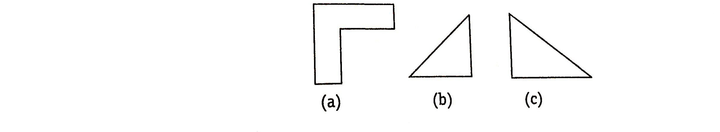Now, if we are asked to form a figure, then there are many possibilities to join these blocks to form a figure. But, we have to analyse the options very carefully such that these three given blocks can be transformed into the figure given in one of the answer options.

If the given options are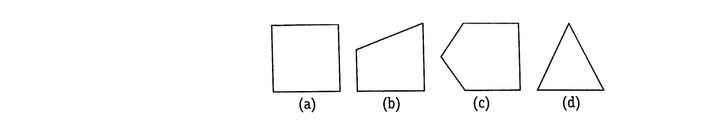The above three blocks can be rearranged as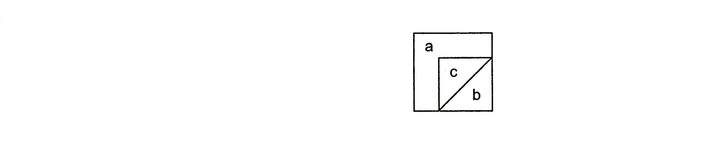So, we have come to the conclusion that figure (a) can be formed from these blocks and hence is the correct answer.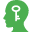Memory Add-ons

1. In the questions based on figure formation, the number of elements given to form a figure must be equal to the elements present in the answer figure. This will help you to easily eliminate some of the option figures.
2. The size of pieces of figures present in the question figure and the size of pieces used to form a figure may vary but their shapes must have to be similar.

Various types of questions based on formation of figures are asked in different competitive exams. Based on their divers nature, we have classified these into several types as follows

TIPS on cracking Non Verbal Reasoning Questions on Formation of Figures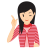Few Important things to Remember

Time is a very important thing in every exams. You should complete your exam within the time frame. But in a competitive exam, question are for testing your calculation ability within a given time frame. They tests, how quickly a student can solve a question paper. This is the reason so many students couldn’t finish their paper within given time. But if you need to solve your government or bank or any other question paper quickly then you should use tips of reasoning and Formation of Figures (Non Verbal Reasoning) which will help you to solve government, bank and other exam papers quickly.

Type - 1 : Based on Arrangement of the Figures

Tip# : In this type of questions, a candidate is required to select a figure, out of the answer choice figures, that can be formed by arranging the pieces given in the question figure.

Following examples will give you a better idea about such type of questions:

Directions (Example Nos. 1-4) In each of the following questions, find the figure out of the answer choice figures, that can be formed by joining the pieces given in the figure

Example 1: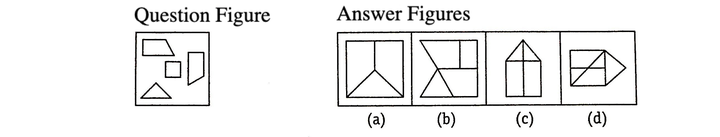Solution: (b) Figure given in option (b) can be formed by joining the pieces given in the question figure as shown below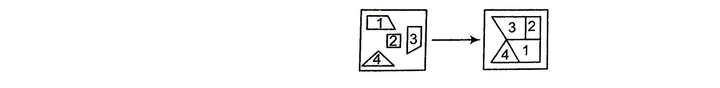Example 2: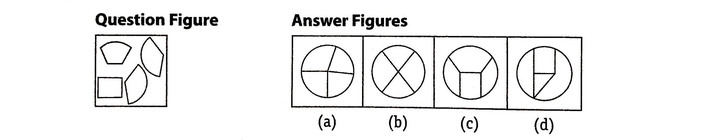Solution: (c) Figure given in option (c) can be formed by joining the pieces given in the question figure as shown below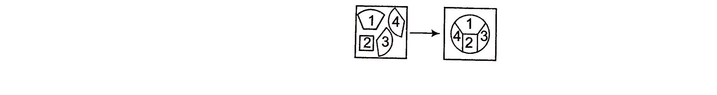Example 3: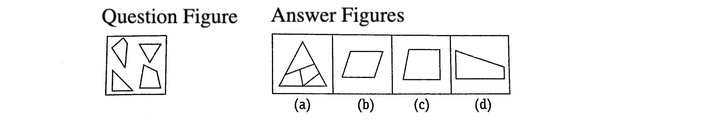Solution: (a) Figure given in option (a) can be formed by joining the pieces given in the question figure as shown below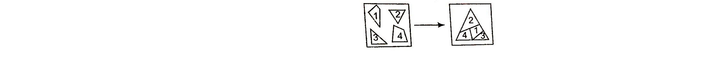Example 4: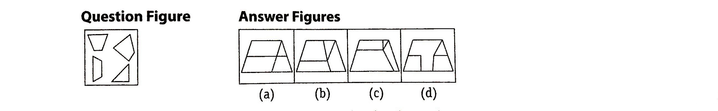Solution: (b) Figure given in option (b) can be formed by joining the pieces given in the question figure as shown below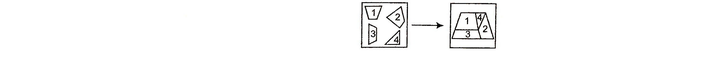Type - 2 : Based on Signs and Symbols

Tip# : Such problems are just the reverse of type - 1 . In this type of questions, question figures are to be formed with the pieces of one of the figures given in the answer choices and the candidate is required to find out the correct answer figure. Sometimes, pieces of boundary lines of the question figure also given in the answer figure, then in this case these pieces are also counted for the formation of question figure. These questions require deep analysis of the question and answer figures.

Directions (Example Nos. 1-2) In each of the following questions, find the figure given in the answer choices, that can form the question figure.

Example 1: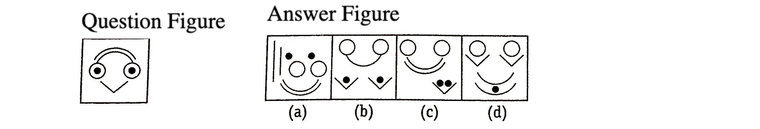Solution: (c) Here, the question figure can be formed by joining the pieces given in option (c) as shown below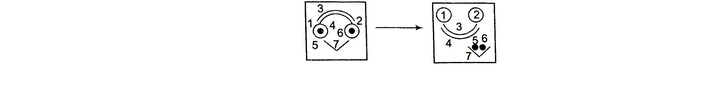Example 2: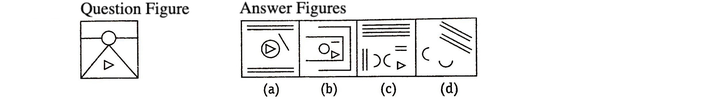Solution: (c) Here, the question figure can be formed by joining the pieces given in option (c) as shown below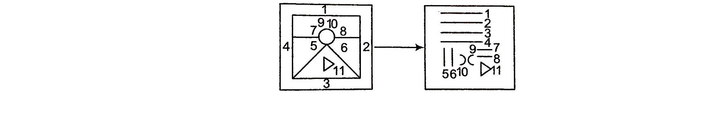Type - 3 : Based on Formation of Triangle

Tip# : In this type of questions, a triangle (equilateral, isosceles etc.) is to be formed by joining three figures out of a group of figures of different designs. A candidate is required to find out the correct combination of figures from the given alternative combinations.

To solve these type of questions, there is no fixed rule or method. These questions requires a deep analysis of various element (design) present to form a figure and spatial analysis of the figure to be formed.

Directions (Example Nos. 1-2) In each of the following questions, five figures are given. Out of them, find the three figures that can be joined to form an equilateral triangle.

Example 1: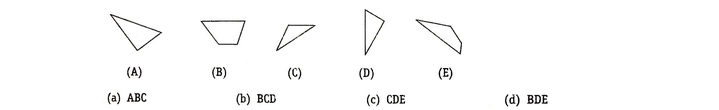Solution: (c) An equilateral triangle has each angle measure equal to 60º and the lengths of all its three sides are also equal, Now, we cannot measure the exact value of angle and sides but we have to closely approximate these measure and values, So, keeping these points in mind, we first combine figure E and C which will look like as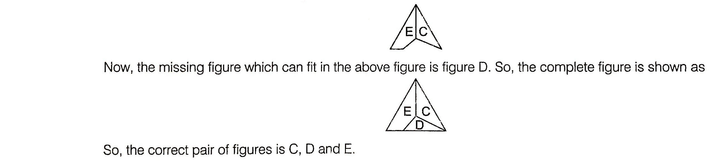Example 2: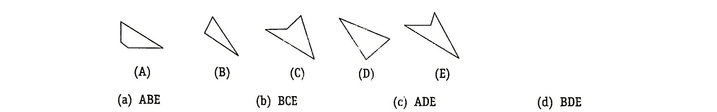Solution: (c) As done in above example, we have to closely approximate the different measures, So first we combine the figure (E) and (A) which will look like as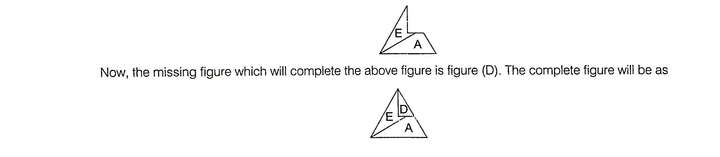Type - 4 : Square Formation Using a Set of Figures

Tip# : In this type of questions, a square is to be formed by joining three figures out of a group of figures of different designs. A candidate is required to find out the correct combination of figures from the given alternative combinations.

Directions (Example Nos. 1-2) In each of the following questions, a set of five figures marked (A), (B), (C), (D) and (E) are given followed by four alternatives (a), (b), (c) and (d). Select the alternative which contains those figures which, if fitted together, will form a complete square.

Example 1: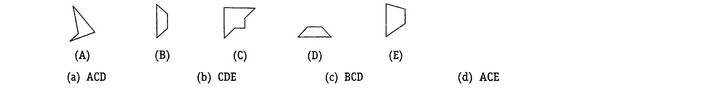Solution: (c) The only figure with right angle is figure (C). If it is fitted with figure (B), the resultant figure will look like the below figure (X).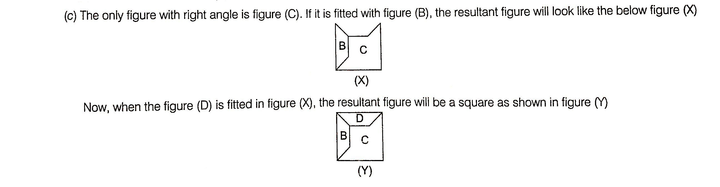Example 2: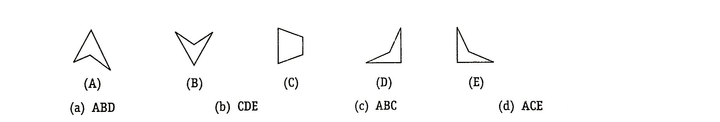Solution: (d) If the figure (C) and (A) are fitted together, the resultant figure will look like the below figure (X).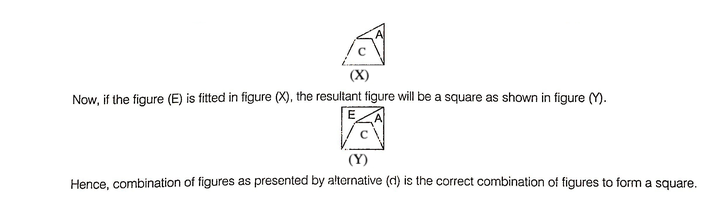LearnFrenzy provides you lots of fully solved Formation of Figures Questions and Answers with explanation.